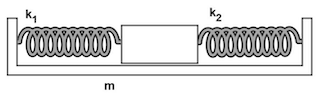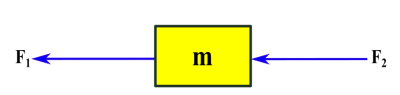# What is the net force acting on the mass (enter first) and what is the effective spring constant?

## Question:The mass m = 11.3 kg shown in the figure is displaced a distance x = 0.149 m to the right from its equilibrium position. k₁ = 117 N/m and k₂ = 205 N/m.

What is the net force acting on the mass (enter first) and what is the effective spring constant?

## Combinations of Springs:

Suppose we have two coil springs, and first, we arrange them in series connection, then we will notice that even if we employ a small force, the system undergoes substantial deformation. This suggests that in a series arrangement, the effective stiffness of the system becomes smaller than the stiffness of the individual springs incorporated in the system. If we arrange the same springs in a parallel connection, the reverse happens, that is, the system becomes stiffer than the individual springs.

Given data:

• {eq}m=\rm 11.3 \ kg {/eq} is the mass attached to the springs.
• {eq}k_1=\rm 117 \ N/m {/eq} is the spring constant of the left spring.
• {eq}k_2=\rm 205 \ N/m {/eq} is the spring constant of the right spring.
• {eq}x=\rm 0.149 \ m {/eq} is the deformation of the springs.The spring force exerted by the left spring is:

{eq}\begin{align} F_1&=k_1x \\[0.3cm] &=\rm 117 \ \frac{N}{m}\times 0.149 \ m \\[0.3cm] &=\rm 17.43 \ N \end{align} {/eq}

The spring force exerted by the right spring is:

{eq}\begin{align} F_2&=k_2x \\[0.3cm] &=\rm 205 \ \frac{N}{m}\times 0.149 \ m \\[0.3cm] &=\rm 30.55 \ N \end{align} {/eq}

The net force acting on the mass is:

{eq}\begin{align} F_{net}&=F_1+F_2 \\[0.3cm] &=\rm 17.43 \ N+30.55 \ N \\[0.3cm] &\simeq \color{blue}{\boxed { \rm 48.0 \ N}} \end{align} {/eq}

Therefore, the net force acting on the mass is {eq}\color{blue}{\boxed { \rm 48.0 \ N}} {/eq}.

The distortion is the same in both springs, so it is nothing but a parallel arrangement of springs. The equivalent or effective spring constant is given by:

{eq}\begin{align} k_{eq}&=k_1+k_2 \\[0.3cm] &=\rm 117 \ \frac{N}{m}+205 \ \frac{N}{m} \\[0.3cm] &= \color{blue}{\boxed { \rm 322 \ N/m}} \end{align} {/eq}

Therefore, the effective spring constant is {eq}\color{blue}{\boxed { \rm 322 \ N/m}} {/eq}.Hooke's Law & the Spring Constant: Definition & Equation

from

Chapter 4 / Lesson 19
201K

After watching this video, you will be able to explain what Hooke's Law is and use the equation for Hooke's Law to solve problems. A short quiz will follow.